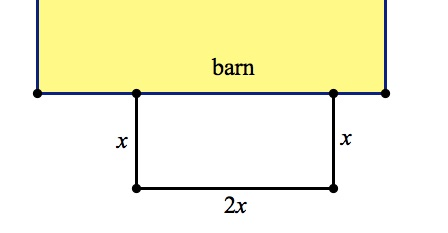An 84 meter length of fencing is attached to the side of a barn in order to fence in a rectangular area, as shown in the figure above. If the length of the side of the fence running perpendicular to the barn is half the length of the side of the fence that is running parallel to the barn, what is the area of the fenced off land?

You know that 84 meters of fencing is used to make 3 sides of a rectangle (the barn forms the fourth side). You also know that one side of the rectangle is twice as long as the other. Let’s call the short side x and the long side 2x.You know that x + x + 2x = 84, so you can solve for x. 4x = 84; x = 21.

That means the rectangle’s width and length will be 21 meters and 42 meters. Its area will therefore be (21 meters)(42 meters) = 882 square meters.